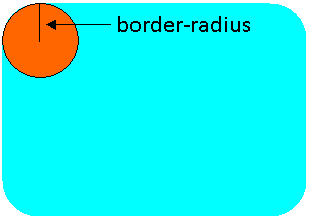The CSS border-radius property is used to set "rounded corners" in an element. The values for this property can either be lengths or percentages. When a length is specified, it represents the length of the radius. When a percentage is specified, it represents the radius as a percentage of the element length.

The picture below shows what border-radius represents in an element with rounded corners.The border-radius property can take one to four values. Below is what each means:

• One value: the radius applies to all four corners.
• Two values: the first radius applies to top-left and bottom-right corners, and the second radius applies to the top-right and bottom-left corners.
• Three values: the first radius applies to the top-left corner, the second radius applies to the top-right and bottom-left corners, and the third radius applies to the bottom-right corner.
• Four values: the values are listed in the following order: top-left, top-right, bottom-right, and bottom-left.

In addition to the convention above, it is also possible to set the border radius for a specific corner. They are accomplished by the following four CSS properties:

For each corner, either one or two values can be defined. When only one value is used, the "rounded corner" looks like a circle. In other words, the x- and y-directions have the same radius. When two values are used, the rounded corner looks like an ellipse (see Example 5 below).

Example 1: Specify a single value

CSS Declaration

which results in the following:

In Example 1, all four corners are rounded with a radius of 30px.

Example 2: Specify two values

CSS Declaration

which results in the following:

In Example 2, the top-left and bottom-right corners have a radius of 30px, and the top-right and bottom-left corners have a radius of 50px.

Example 3: Use percentages

CSS Declaration

which results in the following:

In Example 3, the radius is 50% of the element. Since the element is a square, the result of applying border-radius is a circle. If the element is a rectangle, the result would be an ellipse.

Example 4: Specify each corner individually

CSS Declaration

which results in the following:

In Example 4, the radius is specified for the top-right, top-left, and bottom-left corners. Nothing is specified for the bottom-right corner, so it shows up as a right angle.

Example 5: Set elliptical corners

CSS Declaration

which results in the following:

In Example 5, all four corners are set up as elliptical corners, and all have the same value: 20px for the radius along the x-axis, and 60px for the radius along the y-axis.

Example 6: Set multiple elliptical corners

CSS Declaration